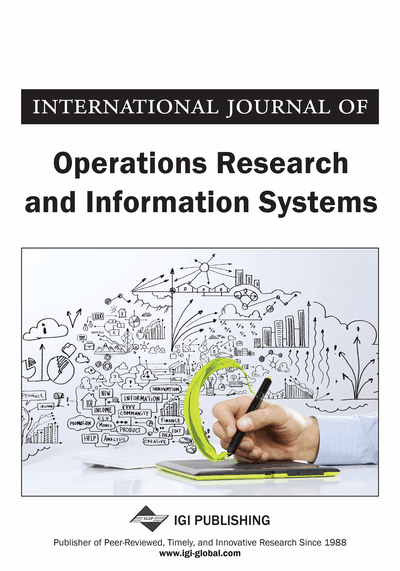# Polynomial Approximation for Two Stage Stochastic Programming with Separable Objective

Lijia Chen (Henan University, China) and Dustin J. Banet (University of Louisville, USA)
DOI: 10.4018/joris.2010070105
Available
\$29.50
No Current Special Offers

## Abstract

In this paper, the authors solve the two stage stochastic programming with separable objective by obtaining convex polynomial approximations to the convex objective function with an arbitrary accuracy. Our proposed method will be valid for realistic applications, for example, the convex objective can be either non-differentiable or only accessible by Monte Carlo simulations. The resulting polynomial is constructed by Bernstein polynomial and norm approximation models. At a given accuracy, the necessary degree of the polynomial and the replications are properly determined. Afterward, the authors applied the first gradient type algorithms on the new stochastic programming model with the polynomial objective, resulting in the optimal solution being attained.
Article Preview
Top

## Introduction

This paper concerns a two stage stochastic programming problem.

(1.1) where,andis a convex function of x for any given ξ∈(Ω,F,P) which is a probability space. We assume E(ξ)<∞ and Var(ξ)<∞. This model has been widely used in the areas of logistics, revenue management, and production control. Consider the following basic properties of the model 1.1. First, the objective

E[g(x,ξ)] is a convex function. Second, when the distribution of ξ is discrete and g(x,ξ) is piecewise convex linear, then E[g(x,ξ)] is a piecewise linear function as well. We can solve the piecewise linear function using its equivalent linear programming model presented in books by Bertsimas and Tsitsiklis (1997), Kall and Wallace (1994). However, a small scale problem can easily lead to computational intractability. For example, when ξ is an eight dimensional random vector and every component is a discrete uniform random variable with 10 evenly likely scenarios, the overall number of scenarios will be 108. Even the resulting model is a sparse Newton system, e.g., linear programming, the modern computer is not able to solve within a timely manner (Nemirovski, 2004). Therefore, the prevailing techniques for stochastic programming models are largely sampling based optimization techniques. For example, the sample average approximation (SAA) method samples certain amount of representative scenarios and solves the reduced problem. The asymptotic optimality and convergence are proved in paper by Shapiro (1989). Another approach is the stochastic approximation (SA) method. Its optimality and convergence can be found in Higle and Sen (1989, 1992). The scenario reduction method is also available for this problem. Both methods are trying to separate all of the scenarios into two categories, the representative scenario and the scenario with extremely low likeliness.

All of these methods have their problems respectively, particularly when the problem scale is fairly large with exponentially growing scenarios. The SAA method and the scenario reduction method actually change the original problem by removing massive scenarios to make the model computational tractable. The optimal solution is actually a random variable and its interval estimation is an on-going research topic. Bayraksan and Morton (2006) proposed a method to assess the solution quality by post-optimization treatment. Determining the sample size pro-actively is yet to be further investigated. The most recent progress on SAA is the Quasi-Monte Carlo techniques by Homem-de-mello (2008). Basically, the author shows that the Quasi-Monte Carlo methods and other variation reduction techniques will improve the speed of convergence. However, the rate of convergence on these methods remains the same which is. The SA method requires evaluating the sub-gradient through sampling. In practice, the estimated sub-gradient may actually penetrate the supporting hyper-plane. In addition, the SA method appears more likely to be slow in convergence. Some special structures may help. If the model 1.1 is simple recourse, it becomes computationally efficient (Birge & Louveaus, 1997; Kall & Wallace, 1994). However, the simple recourse model is an extremely simplified model which rarely appears in business decision.

## Complete Article List

Search this Journal:
Reset
Volume 14: 1 Issue (2023): Forthcoming, Available for Pre-Order
Volume 13: 2 Issues (2022)
Volume 12: 4 Issues (2021)
Volume 11: 4 Issues (2020)
Volume 10: 4 Issues (2019)
Volume 9: 4 Issues (2018)
Volume 8: 4 Issues (2017)
Volume 7: 4 Issues (2016)
Volume 6: 4 Issues (2015)
Volume 5: 4 Issues (2014)
Volume 4: 4 Issues (2013)
Volume 3: 4 Issues (2012)
Volume 2: 4 Issues (2011)
Volume 1: 4 Issues (2010)
View Complete Journal Contents Listing